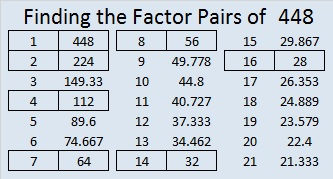# 1623 Easter Basket Challenge Puzzle

### Today’s Puzzle:

Since I’ve recently made puzzles with a pink, purple, or blue Easter egg as well as some blades of grass blowing in the spring wind, it only makes sense that I would also give you an Easter basket in which to hold those other puzzles.

The puzzle is solved if you have written the numbers 1 to 10 in each of the boldly outlined areas of the puzzle, and if those numbers work with the clues to form four multiplication tables.Print the puzzles or type the solution in this excel file: 12 Factors 1614-1623.

If you need a little help, here’s the same puzzle with the factor pairs for the clues written in.And if you want even more help, here’s a 2 1/2 minute video on how to get started. I assume you already know the directions on how to solve this kind of puzzle that I gave at the top of this post.

### Factors of 1623:

• 1623 is a composite number.
• Prime factorization: 1623 = 3 × 541.
• 1623 has no exponents greater than 1 in its prime factorization, so √1623 cannot be simplified.
• The exponents in the prime factorization are 1 and 1. Adding one to each exponent and multiplying we get (1 + 1)(1 + 1) = 2 × 2 = 4. Therefore 1623 has exactly 4 factors.
• The factors of 1623 are outlined with their factor pair partners in the graphic below.

### More About the Number 1623:

1623 is the hypotenuse of a Pythagorean triple:
1023-1260-1623, which is 3 times (341-420-541).

### Today’s Puzzle:

These somewhat tricky level-5 puzzles are probably better suited for middle school and up than younger kids. Use logic on every step and you should be able to find its unique solution.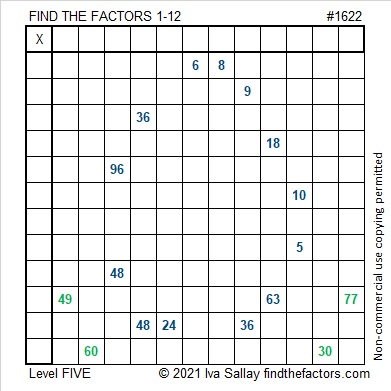Here are some Easter egg puzzles I saw on Twitter. Some are perfect for the littles and others are for older kids. Easter egg hunts can be fun for anyone of any age.

### Factors of 1622:

• 1622 is a composite number.
• Prime factorization: 1622 = 2 × 811.
• 1622 has no exponents greater than 1 in its prime factorization, so √1622 cannot be simplified.
• The exponents in the prime factorization are 1 and 1. Adding one to each exponent and multiplying we get (1 + 1)(1 + 1) = 2 × 2 = 4. Therefore 1622 has exactly 4 factors.
• The factors of 1622 are outlined with their factor pair partners in the graphic below.### More about the Number 1622:

1622 is the sum of four consecutive numbers:
409 + 410 + 411 + 412 = 1622.

# 1621 Give This Purple Egg a Crack!

### Today’s Puzzle:

Here’s a level-5 purple Easter egg for you to try. All you need to do is write the numbers from 1 to 12 in both the first column and in the top row so that those numbers and the given clues function like a multiplication table. Go ahead. Give it a crack!### Factors of 1621:

• 1621 is a prime number.
• Prime factorization: 1621 is prime.
• 1621 has no exponents greater than 1 in its prime factorization, so √1621 cannot be simplified.
• The exponent in the prime factorization is 1. Adding one to that exponent we get (1 + 1) = 2. Therefore 1621 has exactly 2 factors.
• The factors of 1621 are outlined with their factor pair partners in the graphic below.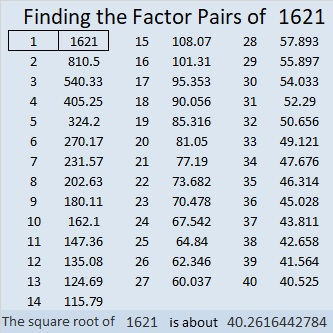How do we know that 1621 is a prime number? If 1621 were not a prime number, then it would be divisible by at least one prime number less than or equal to √1621. Since 1621 cannot be divided evenly by 2, 3, 5, 7, 11, 13, 17, 19, 23, 29, 31, or 37, we know that 1621 is a prime number.

### More about the Number 1621:

OEIS.org informs us that 1621 is in an interesting group of prime numbers.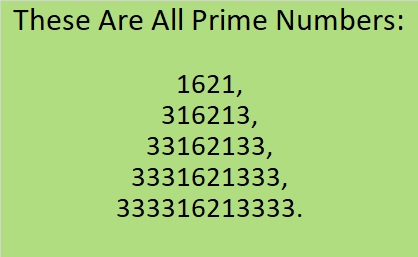I have verified it. They really are all prime!

1621 is the sum of two squares:
39² + 10² = 1621.

1621 is the hypotenuse of a Pythagorean triple:
780-1421-1621, calculated from 2(39)(10), 39² – 10², 39² + 10².

Here’s another way we know that 1621 is a prime number: Since its last two digits divided by 4 leave a remainder of 1, and 39² + 10² = 1621 with 39 and 10 having no common prime factors, 1621 will be prime unless it is divisible by a prime number Pythagorean triple hypotenuse less than or equal to √1621. Since 1621 is not divisible by 5, 13, 17, 29, or 37, we know that 1621 is a prime number.

Occasionally,  we hear that the number of Easter eggs that are found is one or two less than the number of eggs that were hidden. Still most of the time, all the eggs and candies do get found. You really have no trouble finding all those goodies, and the Easter Egg Hunt seems like it is over in seconds.  You can find Easter Eggs but can you find factors? Here’s an Easter Basket Find the Factors 1 – 10 Challenge Puzzle for you. I guarantee it won’t be done in seconds. Can you find all the factors? I dare you to try!Print the puzzles or type the solution in this excel file: 10 Factors 1373-1388

Now I’ll mention a few facts about the number 1377:

• 1377 is a composite number.
• Prime factorization: 1377 = 3 × 3 × 3 × 3 × 17, which can be written 1377 = 3⁴ × 17
• 1377 has at least one exponent greater than 1 in its prime factorization so √1377 can be simplified. Taking the factor pair from the factor pair table below with the largest square number factor, we get √1377 = (√81)(√17) = 9√17
• The exponents in the prime factorization are 4 and 1. Adding one to each exponent and multiplying we get (4 + 1)(1 + 1) = 5 × 2 = 10. Therefore 1377 has exactly 10 factors.
• The factors of 1377 are outlined with their factor pair partners in the graphic below.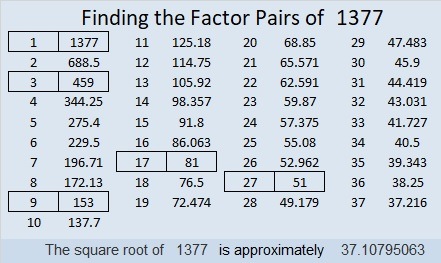1377 is the sum of two squares:
36² + 9² = 1377

1377 is the hypotenuse of a Pythagorean triple:
648-1215-1377 which is (8-15-17) times 81
and can also be calculated from 2(36)(9), 36² – 9², 36² + 9²

# 1038 Hoppy Easter/April Fool’s Day

A trickster Easter bunny left some of my grandchildren candy and other treasures, not in a traditional Easter basket but in pink, green, and orange pumpkins! It’s April Fool’s Day so we shouldn’t be surprised.

That same trickster bunny has a purple pumpkin puzzle for YOU to try today, too. Part of the puzzle is easy while other parts are tricky: Is it 3 or 5 that is the common factor of 30 and 15 that makes this puzzle work?

Hmm…can you figure out where to put the numbers 1 to 10 in the first column and the top row or will you be tricked this April Fool’s Day?Print the puzzles or type the solution in this excel file: 10-factors-1035-1043

Now I’ll tell you a little bit about the number 1038.

1 + 8 is divisible by 3 so 1038 is also divisible by 3. (Including multiples of 3 in the sum isn’t necessary for that divisibility trick to work.)

• 1038 is a composite number.
• Prime factorization: 1038 = 2 × 3 × 173
• The exponents in the prime factorization are 1, 1, and 1. Adding one to each and multiplying we get (1 + 1)(1 + 1)(1 + 1) = 2 × 2 × 2 = 8. Therefore 1038 has exactly 8 factors.
• Factors of 1038: 1, 2, 3, 6, 173, 346, 519, 1038
• Factor pairs: 1038 = 1 × 1038, 2 × 519, 3 × 346, or 6 × 173
• 1038 has no square factors that allow its square root to be simplified. √1038 ≈ 32.218007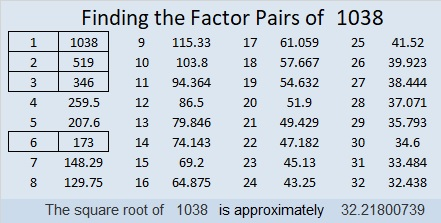1038 is also the hypotenuse of a Pythagorean triple:
312-990-1038 which is 6 times (52-165-173)

# 448 Because He Lives

Happy Easter! The song, “Because He Lives,” is especially appropriate for Easter.

The composer, Sally DeFord, has written some absolutely beautiful music. You can download PDF’s of ANY of her sheet music for FREE on her website. This particular arrangement is written for a duet, but there is also a version for SATB. There are PDF’s of this particular song translated into Spanish, Portuguese, and Finnish. One of the people who commented even gave a translation into Chinese.

Since this is my 448th post, I’ll give some information about the number 448.

448 is another Harshad number because 4 + 4 + 8 = 16, and 448 can be evenly divided by 16.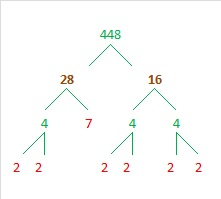• 448 is a composite number.
• Prime factorization: 448 = 2 x 2 x 2 x 2 x 2 x 2 x 7, which can be written 448 = (2^6) x 7
• The exponents in the prime factorization are 6, and 1. Adding one to each and multiplying we get (6 + 1)(1 + 1) = 7 x 2 = 14. Therefore 448 has exactly 14 factors.
• Factors of 448: 1, 2, 4, 7, 8, 14, 16, 28, 32, 56, 64, 112, 224, 448
• Factor pairs: 448 = 1 x 448, 2 x 224, 4 x 112, 7 x 64, 8 x 56, 14 x 32, or 16 x 28
• Taking the factor pair with the largest square number factor, we get √448 = (√64)(√7) = 8√7 ≈ 21.16601